# MATHEMATICS —2004 (Set III— Outside Delhi)

Except for the following questions, ali the remaining questions have been asked in Set I and Set II.

Q. 1. If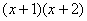is the GCD of the polynomials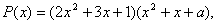andfind the values of a and b.

Ans. (a= -6, b=1)

Q. 2. Using quadratic formula, solve the following quadratic equation for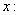Ans.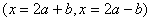Q. 4. The 8 th term of an Arithmetic Progression (A.P.) is 32 and its 12 th term is 52. Find the A.P.

Ans.(-3, 2, 7, 12, ..)

Q. 8. A loan of Rs. 3,900 is to be returned in two equal annual instalments. If the rate of interest is 8% per annum, compounded annually, calculate the amount of each instalment.

Ans. (Rs.2,187)

Q. 14. Construct a triangle ABC in whichand altitude AD = 5.5 cm.

Q. 17. Find the point on the x-axis which is equidistant from the points (-2, 5) and (2, - 3).

Ans. (P (-2,0)

Q. 18. A bag contains 6 red, 5 black and 4 white balls. A ball is drawn from the bag at random. Find the probability that the ball drawn is (i) white (ii) red (iii) not black (iv) red or white.

Ans. (4/15, 2/5, 2/3, 2/3,)

Q. 22. As observed from the top of a light-house, 100 m high above sea level, the angle of depression of a ship, sailing directly towards it, changes from 300 to 600. Determine the distance travelled by the ship during the period of observation. (Use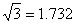)

Ans. (115.46)

Maths 2004 Question Papers Class X
CBSE 2004 Question Papers Class X